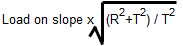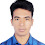# Staircase Design Calculation Details with Excel SheetStaircase Design Calculation Details with Excel Sheet

Hey, I'm Engr. Bala Ram, Today I share wall supported and beam supported staircase design calculation details. I also share this calculation excel spreadsheet.

### How to calculated wall supported staircase design:

Sep - 1: An example Stir riser height R = 6 inches, trade length L = 10 inches (you can change data in excel spreadsheet)

Concrete compressive strength, f'c = 3000 psi.
Yield stress or strength, fy = 60000 psi.

#### Effective Span Length

First landing span, L1 = 3.50 feet.
Waist slab horizontal span, L2 = 7.50 feet.
Second landing span, L3 = 4.00 feet.

Total Horizontal Span, L = L1 + L2 + L3 = 15.00 feet.

#### Thickness of Waist Slab

Waist slab thickness, t = Slab Span / 20 = 9.00 inchs.

Say, slab thickness, t = 7 inches. so,  d = 7-1 = 6 inches.

D.L. of Slab on the slope with landing slab     = (t*12)/150 = 87.50 psf.

D.L. due to Riser ==120.04 psi.
Live load = 100.00 psf, and F.F = 30.00 psf.

Total D.L = 219.54 psf, and L.L = 100.00 psf.

Ultimate Load, w = 1.4*D.L + 1.7*L.L = 477.36 psf.

#### Moment

Moment for Simply Supported Span,#### Shear Check

The factored shear force at a distance "d" from the face of the support:-

### How to calculated Beam supported staircase design:

You can calculate beam supported staircase design. This is the same prosses of wall supported staircase design calculation. In the Excel Spreadsheet, you can full idea, how to design the beam supported staircase.

1.Dami... I liked it

1.Thanks.. keep supporting...

2.3.1.